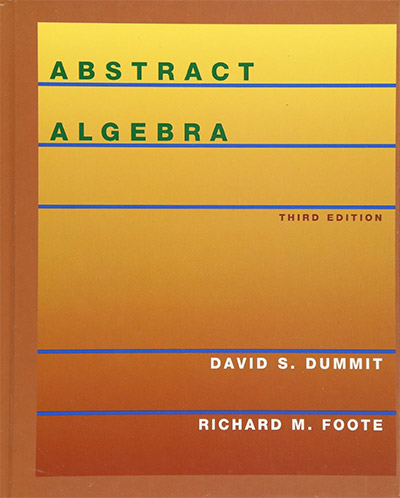# Ideals in Rings

An ideal of a ring is the similar to a normal subgroup of a group. Using an ideal, you can partition a ring into cosets, and these cosets form a new ring - a "factor ring." (Also called a "quotient ring.") After reviewing normal subgroups, we will show you *why* the definition of an ideal is the simplest one that allows you to create factor rings. As an example, we will look at an ideal of the ring Z[x], the ring of polynomials with integer coefficients.

An ideal of a ring is the similar to a normal subgroup of a group. Using an ideal, you can partition a ring into cosets, and these cosets form a new ring - a "factor ring." (Also called a "quotient ring.") After reviewing normal subgroups, we will show you *why* the definition of an ideal is the simplest one that allows you to create factor rings. As an example, we will look at an ideal of the ring Z[x], the ring of polynomials with integer coefficients.

Course Page

## ResourcesAbstract Algebra by Dummit & Foote is a standard textbook used by colleges and universities. It is extremely well written with lots of examples and exercises. It covers all the topics for a solid first course in Abstract Algebra. We use this on occasion as a reference for inspiration or reference. Highly recommended!